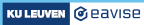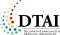3.5. Representing definitions

### 3.5 Representing definitions

A common form of knowledge consists of definitions of concepts. The common way of expressing a definition in FO is by means of an equivalence:

• Definition in natural language: a SOMETHING is called a CONCEPT if and only if it satisfies CONDITION.
• In logic: !x in SOMETHING: CONCEPT(x) <=> CONDITION(x).

### Exercise

In directed graphs, all edges have a direction associated with them that denotes accessibility. For example, if A -> B, B can be reached from A but not vice versa.

One special type of nodes in directed graphs is the sink:

• A node is called a sink if and only if it has no outgoing edges.

Below, we have added this concept to the graph vocabulary. Now add an equivalence to the theory to define it. The theory already contains formulas expressing that all sinks must be coloured in red and all non-sinks in blue, to help visualise the correctness of the definition.

The above exercise shows how definitions are typically represented in FO. However, this tutorial discusses FO(·), which extends FO in a number of ways. One of these extensions is a new way of representing definitions, by means of a special symbol `<-`. This symbol is used as follows (note also the curly brackets):

`{ !x in SOMETHING: DEFINED_CONCEPT(x) <- CONDITION(x). }`

It is of course also possible to have multiple variables if the defined concept takes more than one argument.

For instance, the definition of sink would be:

`{ !x in Node: sink(x) <- ~?y in Node: edge(x,y). }`

When compared to a standard FO equivalence `<=>`, this representation has the following two advantages:

• It makes clear that the purpose of the statement is to provide a definition, whereas an equivalence can also be used to express properties of concepts rather than to define them. For instance, the equivalence `!x[Int] y[Int]: x < y <=> y > x` states a true property of the integers, but does not define anything.
• It captures the inherent directionality of a definition, e.g., it makes clear that sink is defined in terms of edge, instead of the other way around. An equivalence `<=>` has no such direction.

Both these differences change absolutely nothing as far as the models of the statement are concerned: the models of the FO(·) definition are the same as those of the FO equivalence. However, the additional information that is communicated by the use of `<-` can be useful to people reading the formulas, or for software tools that use the formula for some other purpose than computing its models.

There are also a number of other advantages to `<-` that we will discuss later.

###### Made with love (and Hugo) by Joost Vennekens and Simon Vandevelde. :-)